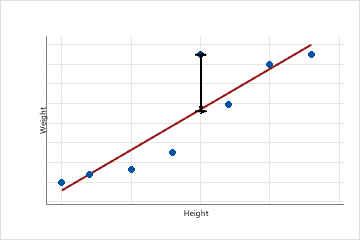# Fits and residuals for Partial Least Squares Regression

Find definitions and interpretation guidance for every statistic in the Fits and residuals table.

## Fits

Fitted values are also called fits or. The fitted values are point estimates of the mean response for given values of the predictors. The values of the predictors are also called x-values.

### Interpretation

Fitted values are calculated by entering the specific x-values for each observation in the data set into the model equation.

For example, if the equation is y = 5 + 10x, the fitted value for the x-value, 2, is 25 (25 = 5 + 10(2)).

## Residual (Res)

A residual (ei) is the difference between an observed value (y) and the corresponding fitted value, (), which is the value predicted by the model.This scatterplot displays the weight versus the height for a sample of adult males. The fitted regression line represents the relationship between height and weight. If the height equals 6 feet, the fitted value for weight is 190 pounds. If the actual weight is 200 pounds, the residual is 10.

### Interpretation

Plot the residuals to determine whether your model is adequate and meets the assumptions of regression. Examining the residuals can provide useful information about how well the model fits the data. In general, the residuals should be randomly distributed with no obvious patterns and no unusual values.

## Standardized residual (SRes)

The standardized residual equals the value of a residual (ei) divided by an estimate of its standard deviation.

### Interpretation

Standardized residuals are useful because raw residuals might not be good indicators of outliers. The variance of each raw residual can differ by the x-values associated with it. This unequal scale causes it to be difficult to assess the sizes of the raw residuals. Standardizing the residuals solves this problem by converting the different variances to a common scale.

## Cross-validated fitted values

In PLS regression, the cross-validated fitted value is the predicted response for each observation in your data set, calculated individually, so the observation can be excluded from the model used to calculate the predicted response for that observation. The cross-validated fitted values are calculated during cross-validation and vary based on how many observations are omitted each time the model is recalculated.

Use cross validated fitted values to identify how well your model predicts data. Cross-validated fitted values are similar to ordinary fitted values, which identify how well your model fits the data.

## Cross-validated residuals

In PLS regression, the cross-validated residuals are the differences between the actual responses and the cross-validated fitted values. The cross-validated residual value varies based on how many observations are omitted each time the model is recalculated during cross-validation.

The residuals measure the model's predictive ability. Minitab uses cross-validated residuals to calculate the PRESS statistic.

By using this site you agree to the use of cookies for analytics and personalized content.  Read our policy